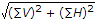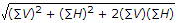# Mechanical Engineering - Engineering Mechanics

### Exercise :: Engineering Mechanics - Section 6

26.

The moment of inertia of a thin rod of mass m and length l,about an axis through its centre of gravity and perpendicular to its length is

 A. ml2/4 B. ml2/ 6 C. ml2/8 D. ml2/12

Explanation:

No answer description available for this question. Let us discuss.

27.

When a body of mass m attains a velocity v from rest in time t, then the kinetic energy of translation is

 A. mv2 B. mgv2 C. 0.5 mv2 D. 0.5 mgv2

Explanation:

No answer description available for this question. Let us discuss.

28.

The unit of angular velocity is

 A. m/min B. rad/s C. revolutions/min D. both (B) and (C) E. none of these

Explanation:

No answer description available for this question. Let us discuss.

29.

If a number of forces are acting at a point, their resultant is given by

 A. (∑V)2 + (∑H)2 B.C. (∑V)2 +(∑H)2 +2(∑V)(∑H) D.Explanation:

No answer description available for this question. Let us discuss.

30.

A force acting in the opposite direction to the motion of the body is called force of friction.

 A. Agree B. Disagree